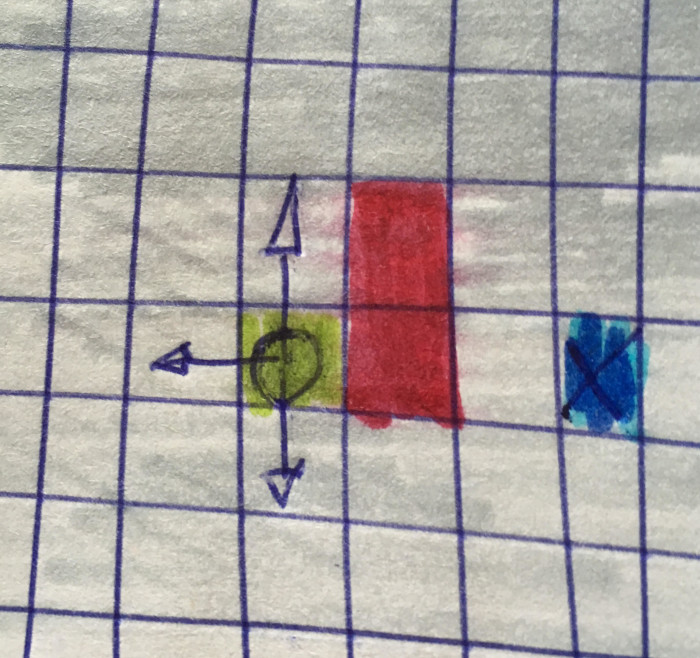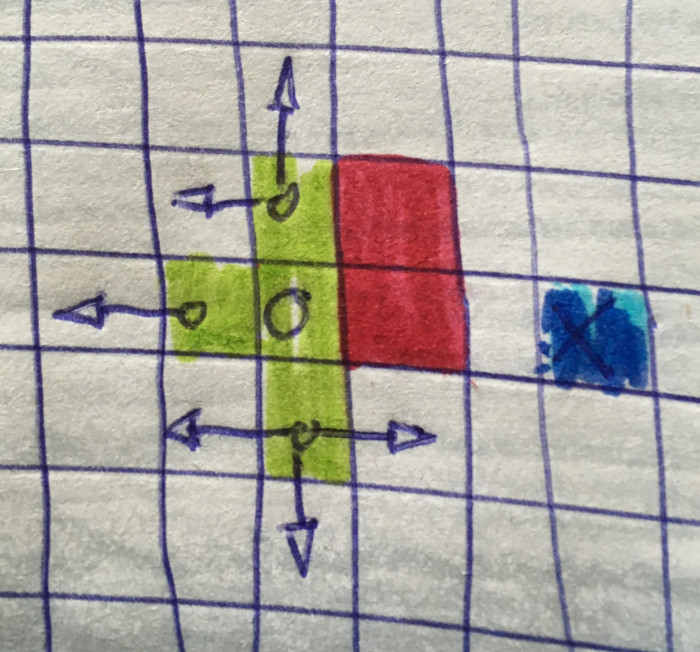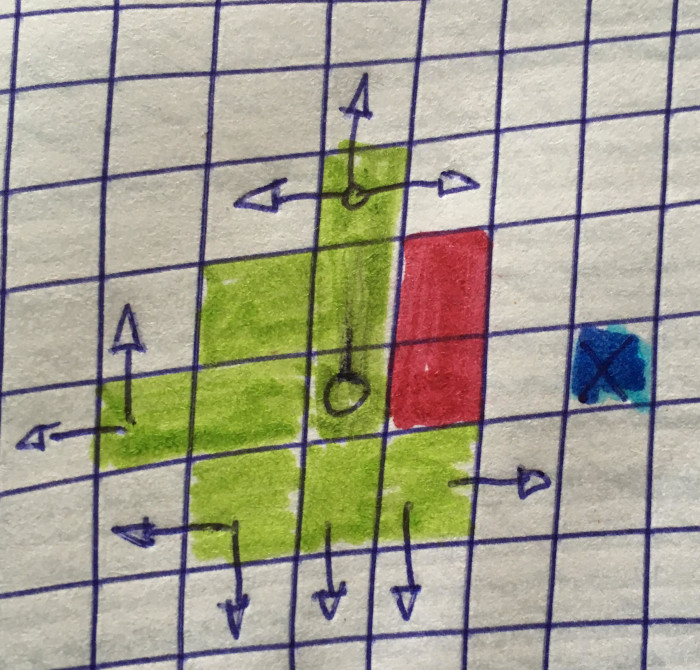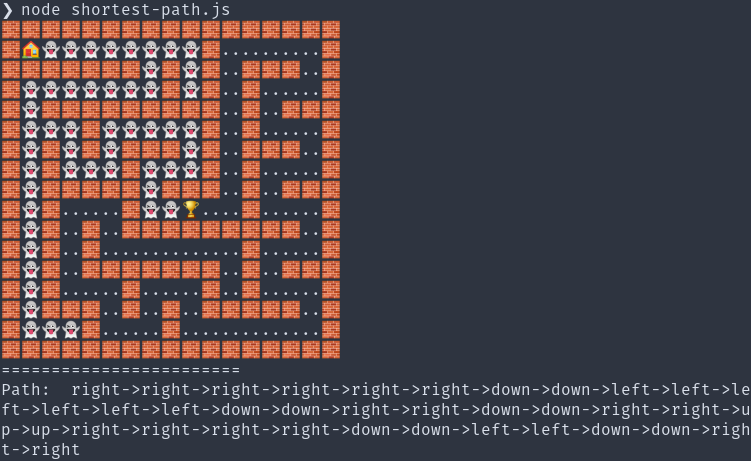# Walk the Maze

In a previous post, we made a simple Depth-first Search algorithm and used it to construct mazes. A logical next step would be to find the shortest path in the type of maze constructed in the previous post. We will accomplish this by the use of Breadth-first Search (BFS). Also, we’ll use the same algorithm in another construct, a more fantasy-like RPG-ish map.

This maze below is made with a Depth-first Search algorithm (the same as before), and we could use a variant of DFS to traverse the ‘tree’, focusing on fixating a path from node A to node B. However, it seem to me that although BFS is more commonly used for tasks like this although DFS, depending on the ‘distance’ and the ‘tree’/map to traverse, would be equally or more efficient.

As mentioned in the post, BFS traverse in one or more directions, ‘level’ by ‘level’. BFS makes no assumptions and the lack heuristics, or some kind of guiding principle.

## Pseudocode

How does this work? Let’s start off with reading the pseudocode for Breadth-first Search from Wikipedia, explain it in general terms and then try to walk through the algorithm, implemented in JavaScript.

## An visual explanation

I believe BFS is better understood by visual means. What’s important is the ‘flux’, how the search colonizes yet another level, examining different parts of the tree.

## #1## #2## #3## #4## A JavaScript implementation

In my console environment this how the result will look like:You have a home, our start location. We’re gooing to find the Trophy (the end point). Every tile we’ve visisted we be marked with a ghost. More interesting, however, is array being returned. The array contains the shortest path between the start and end points.

Our function, `shortestPath` will receive 6 parameters, two included for reasons of clarity (MAP_SIZE_W, MAP_SIZE_H could just as well have been retrieved from the length property row/col of the map array). The essential parameters are `grid` and the two parameters with the x/y positions for start and goal. The parameter named types i also important, we will use it to bootstrap wall/floor tiles.

We will use a library I’ve made, collecting Node.js unicode graphics.

In this case, we check so that the end is ‘walkable’ (not wall) and settles with this (this demo is game-ish and one could presume that the player is on a valid spot. If the endpoints are valid, we set a value for the ending, a Trophy On the other hand, if the point specified for as goal is invalid we return false straight away (the input ‘question’ is invalid). We also set the point where we start as to be represented by a house, our home.

``````function shortestPath(
grid,
startCoordinates,
endCoordinates,
MAP_SIZE_W,
MAP_SIZE_H,
types
) {

if (grid[endCoordinates.y][endCoordinates.x] === types.floor) {
grid[endCoordinates.y][endCoordinates.x] = img.Trophy;
} else return false;

grid[startCoordinates.y][startCoordinates.x] = img.House;``````

We proceed to the next step. We init the queue with a starting location.

``````    // let Q be a queue, label start_v as discovered,
// Q.enqueue(start_v)
let queue = [{
y: startCoordinates.y,
x: startCoordinates.x,
path: [],
status: 'Visited',``````

Now the to the essentials. We will keep traversing until we’ve found our goal destination (until the queue is empty), or have no left to visit (a fail scenario). This demo presumes 4 possible directions to traverse the map/graph. We begin by retrieving the old path (from the queue) and (in the end) stores it for each possible direction if no ‘blocking’ occurs.

We provide the change to be made for each direction in an `Object`. To make our code free from needless conditions we always provide a zero for the opposite spatiality (if we move horizontally, we need to vertical changes and the other way around but we don’t want to check if move left/right or down/up).

Thereafter we have a new state and can thus construct a new location to be examined.

`````` // while Q is not empty
while (queue.length > 0) {

//  v = Q.dequeue()
let currentLocation = queue.shift();

for (let dir of ['up', 'right', 'down', 'left']) {
let newPath = currentLocation.path.slice();
newPath.push(dir);
const possibleDirections = {
up: [0, -1],
right: [1, 0],
down: [0, 1],
left: [-1, 0],
};

let tempX = currentLocation.x + possibleDirections[dir];
let tempY = currentLocation.y + possibleDirections[dir];

let newLocation = {
x: tempX,
y: tempY,
path: newPath,
status: 'Unknown',
};``````

We need to know that this position is within the borders of the 2d array, otherwise, it’s invalid.

Every other possible tile than floor tiles is considered a wall and blocks the path. If the new location isn’t blocked, it’s valid and we set the location as visited and pushed it to the queue. We also mutates (something) you would most likely avoid in a live solution, by replacing the floor tile with a ghost (that’s us).

If the current location is the endpoint (here, the Trophy unicode) we return the path as the final and are done. This path is the shortest possible path between the start and end.

``````

// if v is the goal:
// return v
// for all edges from v to w in G.adjacentEdges(v) do
// if w is not labeled as discovered:
// label w as discovered
// w.parent = v
// Q.enqueue(w)
if ( newLocation.x < 0 || newLocation.x >= MAP_SIZE_W || newLocation.y < 0 || newLocation.y >= MAP_SIZE_H ) {
newLocation.status = ‘Invalid’;
} else if (grid[newLocation.y][newLocation.x] === img.Trophy) {
newLocation.status = ‘Goal’; return {path: newLocation.path, map: grid};
} else if (grid[newLocation.y][newLocation.x] !== types.floor ) {
newLocation.status = ‘Blocked’;
} else {
newLocation.status = ‘Valid’; grid[newLocation.y][newLocation.x] = img.Ghost; queue.push(newLocation);
}
}
}
return false;
}
```
```